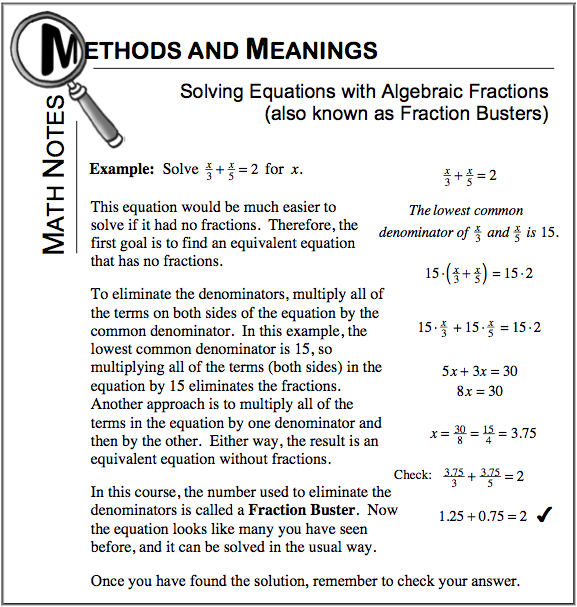Home > ACC7 > Chapter cc27 > Lesson cc27.1.6 > Problem7-70

7-70.
1. Use the Fraction Busters to solve the following problems. Homework Help ✎

1.+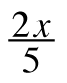=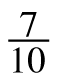2.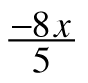+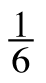=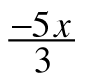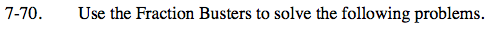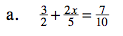Multiply both sides of the equation by a common denominator.

$10\bigg(\frac{3}{2}+\frac{2x}{5}\bigg)=10\bigg(\frac{7}{10}\bigg)$

15 + 4x = 7
4x = −8

x = −2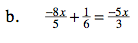See part (a).

$\textit{x}=-\frac{5}{2}=-2\frac{1}{2}\;\;\;\;\;\;\;\;$